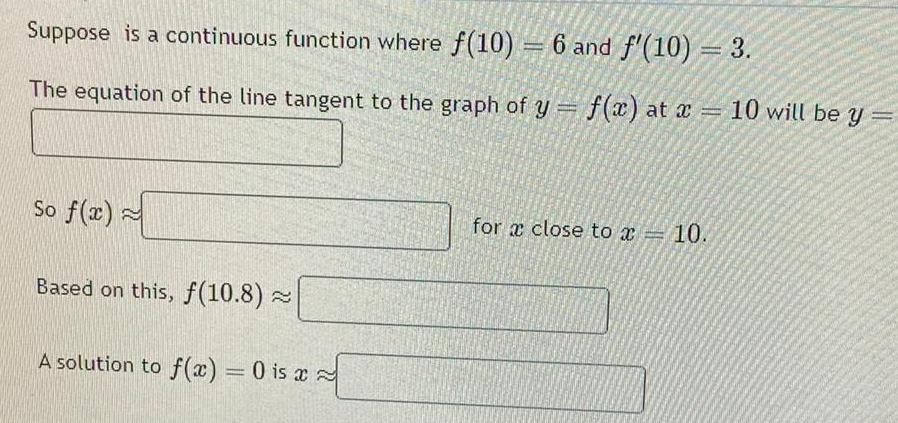Question:

# Suppose is a continuous function where f 10 6 and f 10 3 The

Last updated: 9/19/2023Suppose is a continuous function where f 10 6 and f 10 3 The equation of the line tangent to the graph of y f x at x 10 will be y So f x Based on this f 10 8 A solution to f x 0 is a for a close to a 10